# [Scala MOOC I] Lec1: Functions & Evaluation

[TOC]

In this week, we'll learn the difference between functional imperative programming. We step through the basics of Scala; covering expressions, evaluation, conditionals, functions, and recursion

## 1.1 - Programming Paradigms

imperative programming:

• modify mutable variables
• using assignments
• control structures: if-else, loops, break, continue, return, etc.

~~~> Von Neumann computer: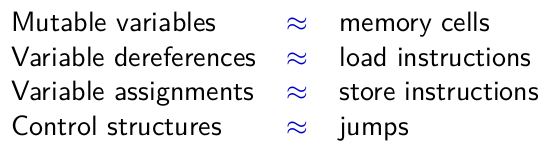conceptualize data structures word-by-word. ⇒ need higher level abstractions (theories).theory doesn't describe mutations!
ex. theories of polynomials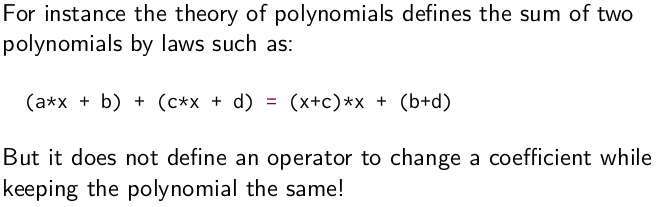To implement high-level concepts following their math theories, there's no place for mutation.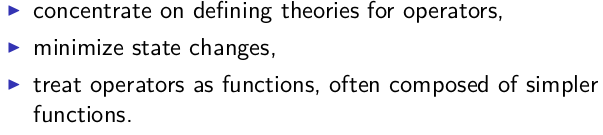Functional programming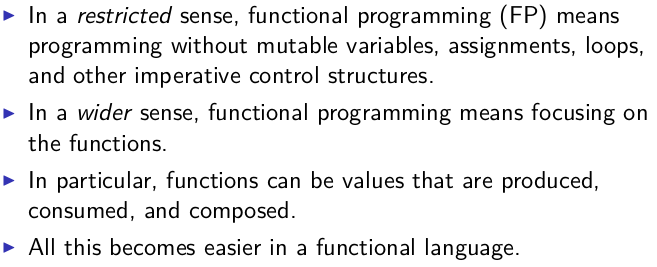functions in FP language are 1st-class citizens.

## 1.2 - Elements of Programming

in a language:

• primitive expressions
• operators to combin expressions
• abstract expressions: introduce a name for an expression

primitive types in scala are written capatilized: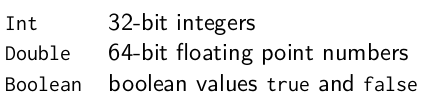### REPL

interactive shell: write expressions and respond its value

### Evaluation

evaluation: expression → value
evaluation stops onces it results in a value.

evaluate non-primitive expressions:

• take the left-most operator
• take (left and right) operands
• apply the operator to operands

evaluate names:
replace the name with the rhs of its definition.

definitions can have parameters.
ex. variable/return types are after their names, seperated by a `:`
`def power(x: Double, y: Int): Double = ...`

evaluate a parameterized function: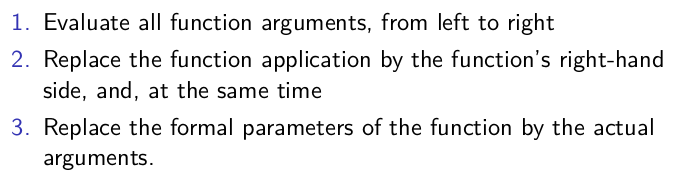This scheme is called "substition model" — formalized in the lambda calculus. This can be applied as long as there is no side effects(ex. modify variable `c++`).

Termination
Q: does every expression evaluates to a value?
NO, ex.
`def loop: Int = loop`

change evaluation strategy
do not reduce argument values before rewrite function application.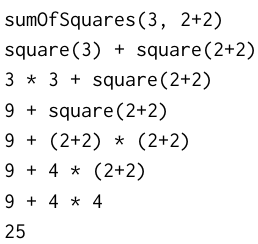## call-by-name and call-by-value

the above evaluation schemes.

• call-by-value: reduce argument values before rewrite function application.

advantage: it evaluates every function argument only once.

• call-by-name: do not reduce arg values

advantage: a function argument is not evaluated if the corresponding parameter is unused in the evaluation
of the function body.

ex.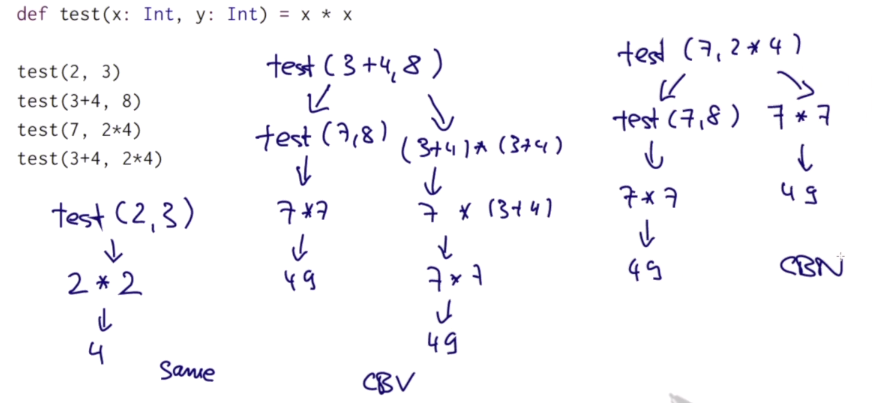## 1.3 - Evaluation Strategies and Termination

CBV and CBN: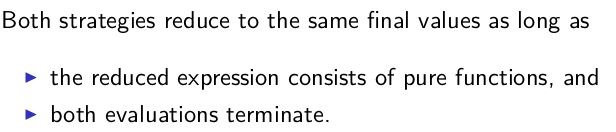ex.

```def first(x: Int, y: Int) = x
first(1, loop)
```

in scala:

• normally use call-by-value (more efficient than call-by-name because it avoid repeated computation of CBN).
• but: if a `=>` before a parameter type, that parameter is called by-name.

ex.

```def constOne(x: Int, y: => Int) = 1
constOne(1+2, loop)
constOne(loop, 1)
```

## 1.4 - Conditionals and Value Definitions

### conditional expressions

the `if-else` expression
the `if-else` is an expression, not statement.
ex. `def abs(x: Int): Int = if (x>=0) x else -x`
the `x>=0` is a boolean expression, sometimes called predicates.

### CBN and CBV for definition

• `def` form is by name, its rhs is evaluated at each use
• `val` is by value, evaluted at the definiton

ex.

```scala> def loop:Boolean = loop
loop: Boolean

scala> def x = loop
x: Boolean

scala> val x = loop
Execution interrupted by signal.
```

exercice: implement `and(x,y)`

```scala> def and(x:Boolean, y: => Boolean) = if(x) y else false
and: (x: Boolean, y: => Boolean)Boolean

scala> and(false, loop)
res3: Boolean = false
```

## 1.5 - Example: square roots with Newton's method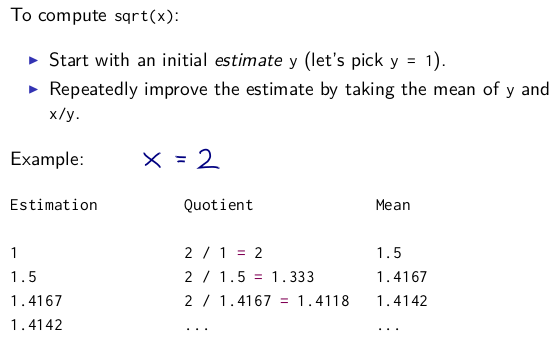`def sqrt(x: Double): Double = ...`

need helper (recursive) functions.

note: in scala the return type of recursive functions must be given.

```def improve(guess: Double, x: Double): Double =
(x / guess + guess) / 2.0

def isGoodGuess(guess: Double, x: Double): Boolean =
math.abs((guess * guess - x)/x)<0.001

def sqrtItr(guess: Double, x: Double): Double = {
if (isGoodGuess(guess, x)) guess
else sqrtItr(improve(guess, x), x)
}

def sqrt(x:Double): Double = sqrtItr(1, x)
```

## 1.6 - Blocks and Lexical Scope

### nested functions

put aux functions inside a function.

```def sqrt(x:Double): Double = {
def improve(guess: Double, x: Double): Double =
(x / guess + guess) / 2.0

def isGoodGuess(guess: Double, x: Double): Boolean =
math.abs((guess * guess - x)/x)<0.001

def sqrtItr(guess: Double, x: Double): Double = {
if (isGoodGuess(guess, x)) guess
else sqrtItr(improve(guess, x), x)
}

sqrtItr(1, x)
}
```

### blocks and visibility

blocks: delimited by braces `{...}`
The last element of a block is an expression that defines its value.
Blocks are themselves expressions; a block may appear everywhere an expression can.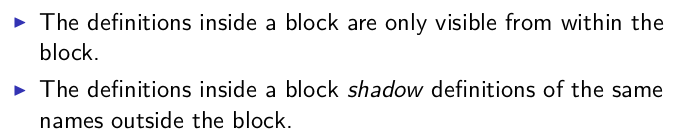ex.

```val x = 0
def f(y: Int) = y +1
val result = {
val x = f(3);
x * x
} + x // result = 16
```

ex. eliminate the parameter `x` of helper functions inside the `sqrt` function

```def sqrt(x:Double): Double = {
def improve(guess: Double): Double =
(x / guess + guess) / 2.0

def isGoodGuess(guess: Double): Boolean =
math.abs((guess * guess - x)/x)<0.001

def sqrtItr(guess: Double): Double = {
if (isGoodGuess(guess)) guess
else sqrtItr(improve(guess))
}

sqrtItr(1)
}
```

about multiline expressions: put the operator in the first line to avoid ambguity.## 1.7 - Tail Recursion

review: evaluation of function application.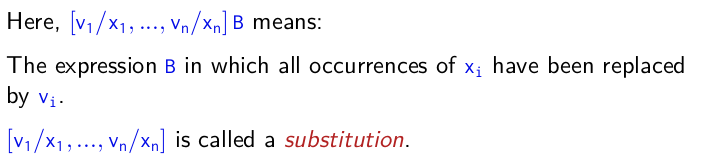ex1. gcd

```def gcd(a:Int, b:Int): Int=
if(b==0) a else gcd(b, a%b)
```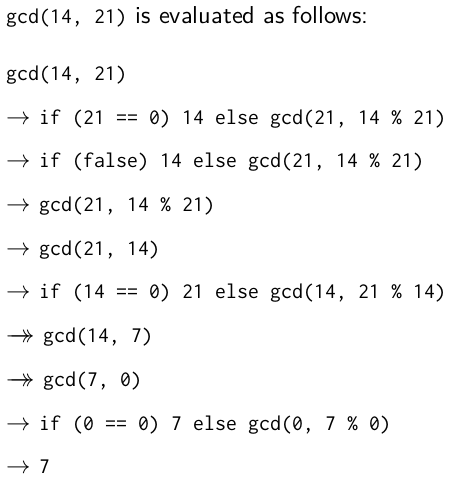ex2. factorial

```def factorial(n:Int): Int =
if(n==0) 1 else n*factorial(n-1)
```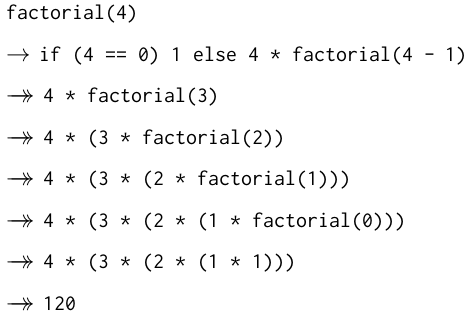difference from the `gcd` case:

• in `gcd`, the expression is reduced to a `gcd()`
• in `factorial`, add one more element to the expression in each step!

### tail recursion

if a function calls itself as its last action, then the function's stack can be reused.
⇒ tail recursion functions are iterative process.

In general, if the last action of a function consists of calling a function (which may be the same), one stack frame would be sufficient for both functions. Such calls are called tail-calls.

tail-recursive function:

• more efficient
• can avoid stackoverflow
• might not be as clear as a non-tailrec version

add `@tailrec`annotation to the function, if its not tailrec, an error will be shown.

```@tailrec
def gcd(a: Int, b: Int): Int = ...
```

exercice: turn factorial into tailrec fashion.
→ idea: use an accumulator (partial result) `acc` .

```def factorialTR(n:Int):Int = {
@tailrec
def fact(n:Int, acc:Int):Int = {
if(n==0) acc
else fact(n-1, n*acc)
}
fact(n, 1)
}
```

## Programming Assignment: Recursion

```  /**
* Exercise 1
*/
def pascal(c: Int, r: Int): Int = {
if (c == 0 || c == r) 1
else pascal(c, r - 1) + pascal(c - 1, r - 1)
}

/**
* Exercise 2
*/
def balance(chars: List[Char]): Boolean = {
def balance_rec(chars: List[Char], acc: Int): Boolean = {
if (acc<0) false
else if (chars.isEmpty) acc == 0
else if (chars.head == '(') balance_rec(chars.tail, acc+1)
else if (chars.head == ')') balance_rec(chars.tail, acc-1)
else balance_rec(chars.tail, acc)
}

balance_rec(chars, 0)
}

/**
* Exercise 3
*/
def countChange(money: Int, coins: List[Int]): Int = {
if(money==0 ) 1
else if(coins.isEmpty || money<0) 0
else countChange(money, coins.tail) + countChange(money-coins.head, coins)
}
```

#### Part 2 of series «Functional Programming Principles in Scala»：

comments powered by Disqus